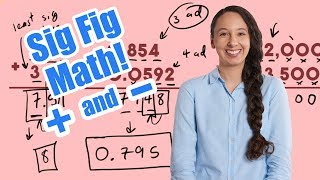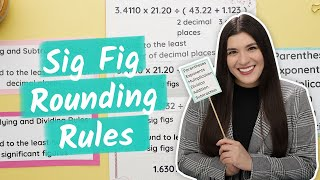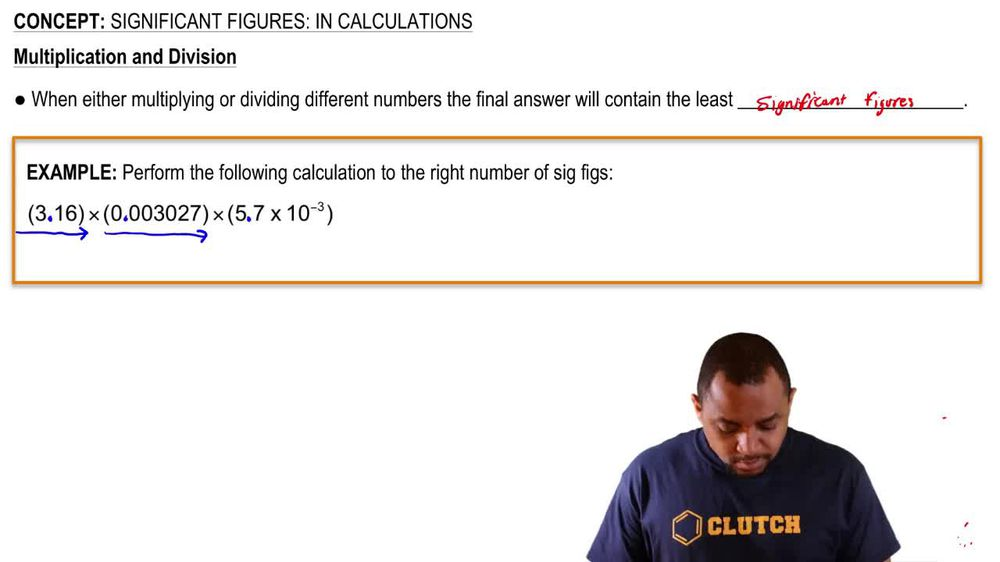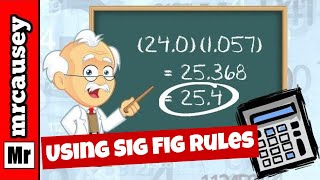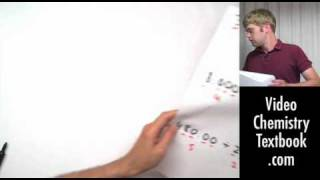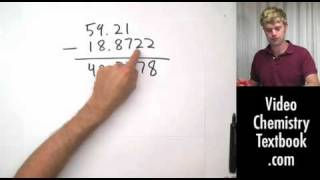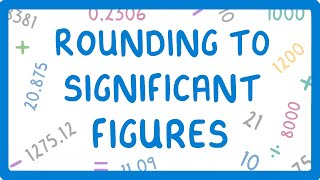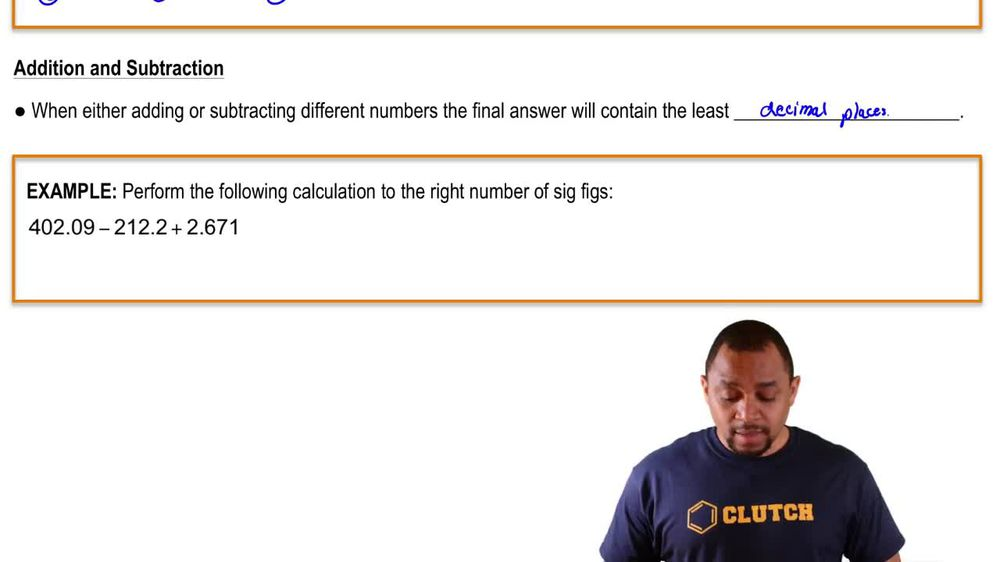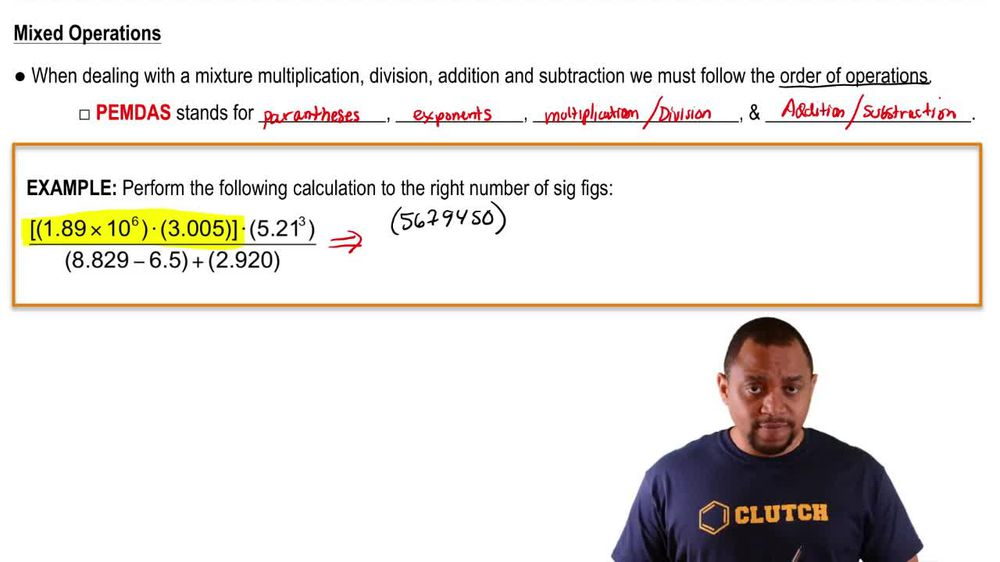Start typing, then use the up and down arrows to select an option from the list.
1. 1. Intro to General Chemistry2. Significant Figures: In Calculations
Problem

# Express the results of the following calculations with the correct number of significant figures. (d) 5502.3 + 24 + 0.01

Relevant Solution1m
Play a video:
Hi everyone today we have a question asking us to perform the following operation and we need to express the answer using the correct number of significant figures. We have 0.01 plus 0. And that equals 0.06871. And now we need to remember our rules for addition and subtraction using significant figures and that is the least number of decimal points. So let's look at our decimal points here. We have two for this number and we have five for this number. So we're going to go with two. So that means we need to look at our six here And we need to look to the right of R6 to see if we need to round up if it is five or above. We need to round up And it is eight so it is five or above. That means our answer is 0.07. Thank you for watching. Bye.